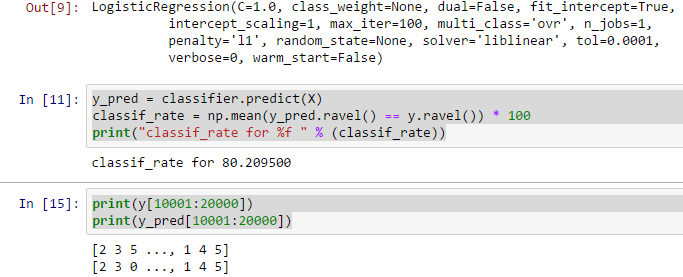Liam

23 Apr, 2016## Summary of 1_notmnist

Basically 1_notmnist is to learn how to display data in Jupyter Notebook. Besides, it also let us know on sklearn - a python machine library - so that we can then compare with TensorFlow. This is the exact ipynb file at Tensorflow Github Repo.

## Notice

This is as a form of sharing and discuss on better way to solve 1_notmnist problem. Do not copy and paste directly as it does not help on improving yourself + the answer is not optimized.

The entire series of TensorFlow Udacity can be found at tensorflow-udacity tag

## Solving Problem 6

``````def reshape(a):
return a.reshape(a.shape,a.shape*a.shape)
y = t['train_labels']
X = reshape(t['train_dataset']) # reshape it to 2d array
del(t) # this should free up more memory spaces
# choose form 0:10000 because not enough memory for the docker
# probably a way to do batch learning with scikit-learn
# http://scikit-learn.org/stable/modules/generated/sklearn.linear_model.LogisticRegression.html
# http://scikit-learn.org/stable/auto_examples/classification/plot_classification_probability.html
C = 1.0
classifier = LogisticRegression(C=C, penalty='l1')
classifier.fit(X[0:10000], y[0:10000])
y_pred = classifier.predict(X)
classif_rate = np.mean(y_pred.ravel() == y.ravel()) * 100
print("classif_rate for %f " % (classif_rate))
# we now see how it is predicted using sample 10001 to 20000, which is not used for training
# actually should calculate the accuracy in percentage.
print(y[10001:20000])
print(y_pred[10001:20000])
``````## Comment

This time we have learnt how to use scikit-learn to do LogisticRegression for notMNIST. As we can see, the classification accuracy is still not bad.

I will expect that tensorflow is either faster and more structured compared to this solution (or google probably will not use this as an example)